Quiz on exterior angles of trianglesOne Day Only Black Friday Sale: Get 30% OFF All Diplomas! Sale only on Friday, 27th November 2020### This Course has been revised!

For a more enjoyable learning experience, we recommend that you study the mobile-friendly republished version of this course.

Take me to revised course.

- or -

Continue studying this course

# Quiz on exterior angles of triangles

• Study Reminders

We'll email you at these times to remind you to study

You can set up to 7 reminders per week

#### You're all set

We'll email you at these times to remind you to study

Monday

Tuesday

Wednesday

Thursday

Friday

Saturday

Sunday

• Study Notes
• Discuss This Topic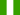Aminu B. 1 0 I Need Help on EXTERIOR ANGLES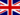Sarah H. 1 0 I'm certain that I have answered them all correctly but it is not turning green. 1 - 60 + 40 = 100 (interior opposites = exterior) a=100 2 - Isosceles so 50 + 50 = 100 180-100 = 80 180-80 = 100 a= 100 3 - 180 - 145 = 35 90+35 = 125 180-125 = 55 a = 55 4 - 180 - 120 = 60 60+90 = 150 180 - 150 = 30 180 - 30 = 150 a = 150 5 - 180 - 120 = 60 180-140 = 40 60 + 40 = 100 180 - 100 = 80 a = 80 Can anyone tell me where I have gone wrong please ?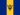Alisa G. 0 0 The Quiz on exterior angles,i cannot access itDamarco W. 6 0 I have done two quiz's and can't submit them because there is no submit button. How are they to be graded?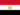Ahmed W. 0 0 The Quiz on exterior angles of triangles is not workRebecca C. 0 0 module exterior angle equals the sum of the two opposite interior angles topic 60+40 =100 then 50+50(isosceles )=100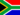Nkululeko M. 1 0 module exterior angle equals the sum of the two opposite interior angles topic 60+40 =100 then 50+50(isosceles )=100Ana Rita M. 0 0 100 both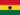Maxwell D. 0 0 Critical to solving Triangle problems. Exterior angle is equal to the sum of opposite angles inside a Triangle.
• Text Version